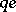# Massless Klein-Gordon equation

\begin{equation*} 0 = \left[ - \left( \frac { \partial } { \partial t } - i \frac { q e } { \hbar } \phi \right) ^ { 2 } + \right. \end{equation*}

\begin{equation*} \left.+ c ^ { 2 } \left( \nabla - i \frac { q e } { \hbar c } A \right) ^ { 2 } + \frac { c ^ { 4 } m ^ { 2 } } { \hbar ^ { 2 } } \right] \psi ( t , \mathbf{x} ) \end{equation*}

for the case where the mass parameter $m$ is equal to zero. The constant $c$ stands for the speed of light, $e$ is the charge of the positron, $\hbar = h / 2 \pi$ where $h$ is the Planck constant, $( t , \mathbf{x} )$ are the time, respectively space, variables, and $i$ is the imaginary unit. The (complex-valued) solution $\psi$ describes the wave function of a relativistic spinless and massless particle with chargein the exterior electro-magnetic field $( \phi , \mathbf{A} )$. It is a second-order, hyperbolic partial differential equation. Solutions are being studied in, e.g., [a4], [a5].

If the outer field is zero, $( \phi , \mathbf{A} ) = 0$, or the coupling of the spin to the magnetic potential $\mathbf{A}$ can be neglected, the massless Klein–Gordon equation also can be used for the description of massless spin-carrying particles, such as e.g. photons. In the case without outer fields the massless Klein–Gordon equation becomes equivalent to the wave equation with wave speed $c$ and is independent of the magnitude of Planck's constant $h$. This explains, why the wave nature of massless particles, such as e.g. photons ( "light" ), can also be observed on a macroscopic scale — in contrast with the wave nature of massive particles (cf.also Massless field; Massive field).

The interpretation of the wave function $\psi$ as a quantum mechanical "probability amplitude" (similarly as in the case of the Schrödinger equation), however, is not consistent, since the quantity $\int _ { \mathbf{R} ^ { 3 } } | \psi ( t , \mathbf{x} ) | ^ { 2 } d \mathbf{x}$ in general depends on the time parameter $t$. Furthermore, the existence of negative frequency solutions is in contrast with the required lower boundedness of the energy ( "stability of matter" ). These problems are resolved through a re-interpretation of $\psi ( t , \mathbf{x} )$ as a quantum field (cf. Quantum field theory), see e.g. [a6], [a7].

In recent time (as of 2000) solutions of the Klein–Gordon equation on Lorentzian manifolds have attracted increasing attention in connection with the theory of quantized fields on curved space-time, cf. e.g. [a8].

How to Cite This Entry:
Massless Klein-Gordon equation. Encyclopedia of Mathematics. URL: http://encyclopediaofmath.org/index.php?title=Massless_Klein-Gordon_equation&oldid=50673
This article was adapted from an original article by S. AlbeverioH. Gottschalk (originator), which appeared in Encyclopedia of Mathematics - ISBN 1402006098. See original article